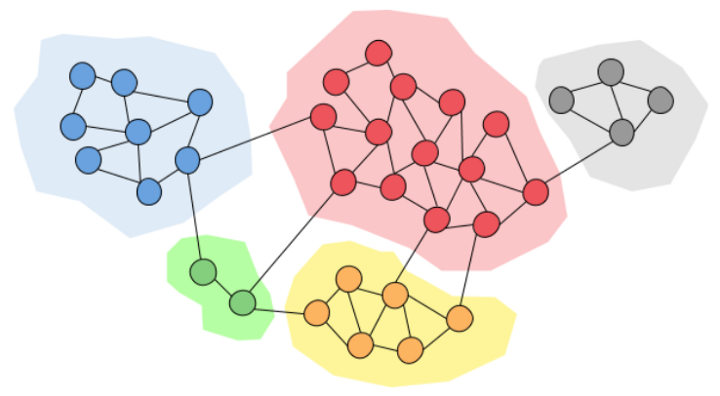PyCSP3
COP  easy

# Problem Community Detection

The problem is to partition the set of nodes of a graph (the parts forming so-called communities) while seeking maximum modularity (as defined by a matrix). Among possible constraints related to some background knowledge, one can impose that some pairs of nodes must be assigned to the same or different communities. The problem of constrained community detection is described with many details in “A declarative approach to constrained community detection” by M. Ganji, J. Bailey, and P. Stuckey, in proceedings of CP’17.

Forming Communities. Image by Thamindu Dilshan JayawickramafromTo build a COP (Constraint Optimization Problem) model, we need first to import the library PyCSP$^3$:

from pycsp3 import *


Then, we need some data. The graph is given by its adjacency matrix, and nodes that must be put together or in separate communities are indicated by lists. The maximum number of communities is also indicated.

graph = [
[0,0,1,0,0,1],
[0,1,0,0,0,0],
[0,0,0,1,0,0],
[0,0,1,0,0,0],
[0,0,1,0,1,0],
[1,0,1,0,1,1]]
together = [[0,5], [2,3], [4,5]]
separate = [[1,3], [3, 5]]
m = 3  # max communities
n = len(graph)


We compute the modularity matrix W:

def modularity_matrix():
degrees = [sum(graph[i]) for i in range(n)]  # node degrees
sum_degrees = sum(degrees)  # mu ltiplier used to avoid fractions
return [[sum_degrees * graph[i][j] - degrees[i] * degrees[j] for j in range(n)]
for i in range(n)]

W = modularity_matrix()


We can display it:

print(W)

[[-4, -2, 9, -2, -4, 3], [-2, 10, -1, -1, -2, -4], [-2, -1, -1, 10, -2, -4], [-2, -1, 10, -1, -2, -4], [-4, -2, 9, -2, 7, -8], [3, -4, 7, -4, 3, -5]]


We start our COP model by introducing an array $x$ of variables.

# x[i] is the community of the ith node
x = VarArray(size=n, dom=range(m))


We can display the structure of the array, as well as the domain of the first variable (note that all variables have the same domain).

print("Array x: ", x)
print("Domain of any variable: ", x.dom)

Array x:  [x, x, x, x, x, x]
Domain of any variable:  0..2

Concerning the constraints, we have to post two groups of constraints [Intension](/documentation/constraints/Intension): the first one to ensure that some nodes belong to the same community, and the second one tp ensure that some nodes belong to separate communities.

satisfy(
# considering nodes that must belong to the same community
[x[i] == x[j] for i, j in together],

# considering nodes that must not belong to the same community
[x[i] != x[j] for i, j in separate]
);


We can display the internal representation of the two posted constraints; this way, although a little bit technical, we can see that the arguments of the two constraints are correct.

print(posted())

intension(function:eq(x,x))
intension(function:eq(x,x))
intension(function:eq(x,x))
intension(function:ne(x,x))
intension(function:ne(x,x))


Interestingly, by calling the function solve(), we can check that the problem is satisfiable (SAT). We can also display the found solution. Here, we call the function values() that collects the values assigned to a specified list of variables.

if solve() is SAT:
print(values(x))

[0, 0, 1, 1, 0, 0]


We can display all solutions, as follows.

if solve(sols=ALL) is SAT:
for i in range(n_solutions()):
print(f"Solution {i+1}: {values(x, sol=i)}")

Solution 1: [0, 0, 1, 1, 0, 0]
Solution 2: [0, 0, 2, 2, 0, 0]
Solution 3: [0, 1, 2, 2, 0, 0]
Solution 4: [0, 2, 1, 1, 0, 0]
Solution 5: [2, 2, 1, 1, 2, 2]
Solution 6: [2, 0, 1, 1, 2, 2]
Solution 7: [2, 1, 0, 0, 2, 2]
Solution 8: [2, 2, 0, 0, 2, 2]
Solution 9: [1, 2, 0, 0, 1, 1]
Solution 10: [1, 1, 0, 0, 1, 1]
Solution 11: [1, 1, 2, 2, 1, 1]
Solution 12: [1, 0, 2, 2, 1, 1]


One way of breaking symmetries is to post a constraint Precedence.

satisfy(
# tag(symmetry-breaking)
Precedence(x)
);


This guarantees that if 2 is assigned, in the list $x$, it is preceeded by 1, and if 1 is assigned, it is preceeeded by 0. Actually, the code above is equivalent to:

satisfy(
# tag(symmetry-breaking)
Precedence(x, values= [0,1,2])
);


Now, we can check that only 2 solutions remains:

if solve(sols=ALL) is SAT:
for i in range(n_solutions()):
print(f"Solution {i+1}: {values(x, sol=i)}")

Solution 1: [0, 0, 1, 1, 0, 0]
Solution 2: [0, 1, 2, 2, 0, 0]


Finally, we can post the objective:

maximize(
Sum((x[i] == x[j]) * W[i][j] for i, j in combinations(n, 2) if W[i][j] != 0)
);


We can display the internal representation of the objective to check if everything looks good:

print(objective())

maximize(sum(list:[eq(x,x), eq(x,x), eq(x,x), eq(x,x), eq(x,x), eq(x,x), eq(x,x), eq(x,x), eq(x,x), eq(x,x), eq(x,x), eq(x,x), eq(x,x), eq(x,x), eq(x,x)], coeffs:[-2, 9, -2, -4, 3, -1, -1, -2, -4, 10, -2, -4, -2, -4, -8]))


We can run the solver, with this optimization task. Note that we need to check that the status returned by the solver is now OPTIMUM.

if solve() is OPTIMUM:
print("assignment: ", values(x))
print(f"objective value: {bound()}")

assignment:  [0, 1, 2, 2, 0, 0]
objective value: 1


Finally, we give below the model in one piece. Here the data is expected to be given by the user (in a command line).

from pycsp3 import *

graph, together, separate, m = data  # m is the maximum number of communities
n = len(graph)  # number of nodes

def modularity_matrix():
degrees = [sum(graph[i]) for i in range(n)]  # node degrees
sum_degrees = sum(degrees)  # multiplier used to avoid fractions
return [[sum_degrees * graph[i][j] - degrees[i] * degrees[j] for j in range(n)]
for i in range(n)]

W = modularity_matrix()

# x[i] is the community of the ith node
x = VarArray(size=n, dom=range(m))

satisfy(
# considering nodes that must belong to the same community
[x[i] == x[j] for i, j in together],

# considering nodes that must not belong to the same community
[x[i] != x[j] for i, j in separate],

# tag(symmetry-breaking)
Precedence(x)
)

maximize(
Sum((x[i] == x[j]) * W[i][j] for i, j in combinations(n, 2) if W[i][j] != 0)
)